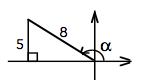### Home > PC > Chapter 6 > Lesson 6.2.1 > Problem6-73

6-73.
1. Use the diagram below to find exact values of the following trig expressions. Homework Help ✎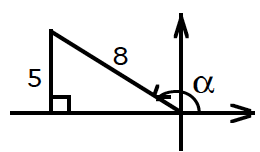1. sin α

2. cos α

3. tan α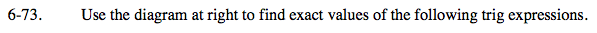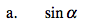Use the values given in the diagram.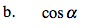Calculate the length of the base of the triangle. What should the sign be?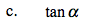Use the information in the triangle and your calculated base from part (b).